Angles made by the transversal - Questions

Suppose we are given,

two lines & a transversal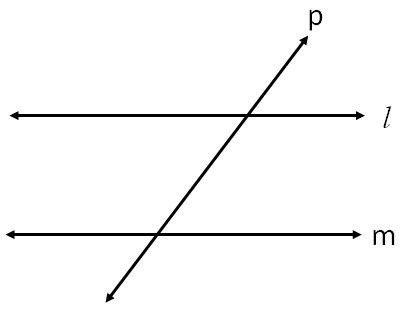We know that

For parallel lines

• Corresponding angles are equal
• Alternate interior angles are equal
• Interior angles on same side of transversal is supplementary
• Alternate exterior angles are equalBut the opposite is true as well

• If corresponding angles are equal,
Line are parallel

• If alternate interior angles are equal,
lines are parallel

• If sum of interior angles on same side of transversal is 180°,
lines are parallel.

• If alternate exterior angles are equal,
lines are equal.

Let’s do some questions

Is l m ?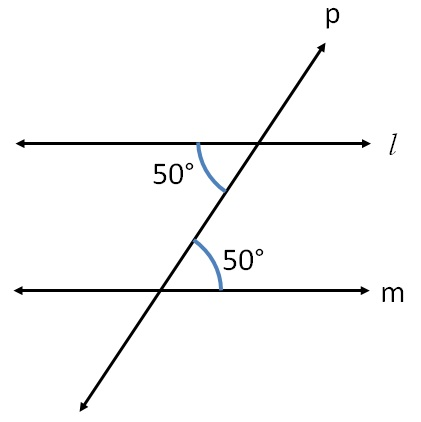-a-

Here,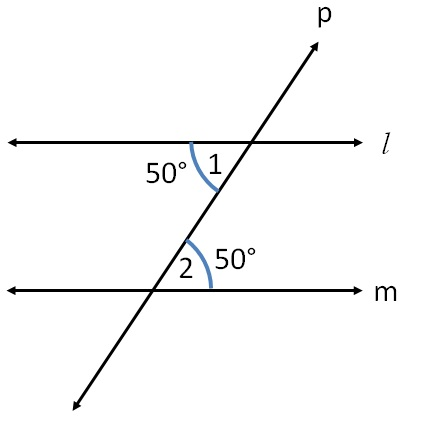Here,

∠1 = ∠2 = 50°

For lines l & m,

With transversal p

∠1 & ∠2 are alternate interior angles.

And they are equal.

So, lines l & m are parallel

-ea-

Is l m ?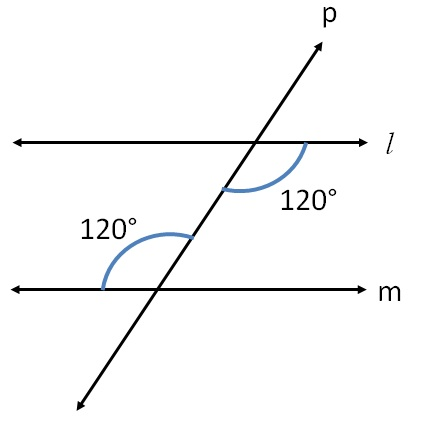-a-

Here,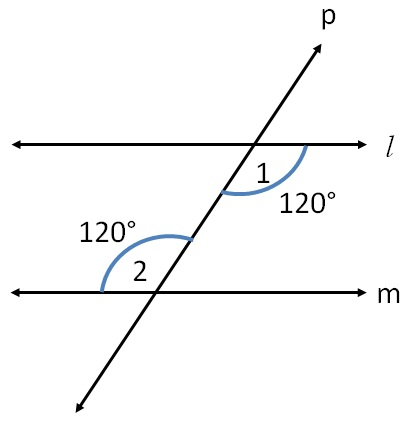Here,

∠1 = ∠2 = 120°

For lines l & m,

With transversal p

∠1 & ∠2 are alternate interior angles.

And they are equal.

So, lines l & m are parallel

-ea-

Is l ∥ m ?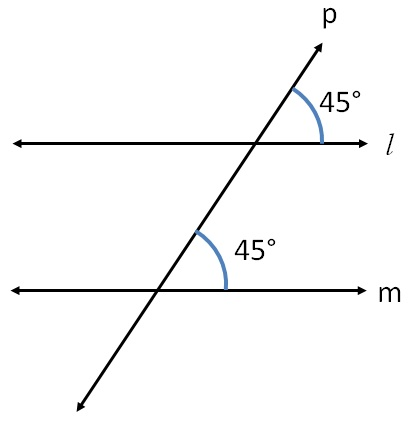-a-

Here,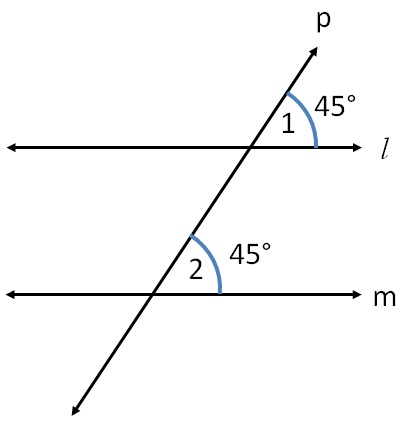Here,

∠1 = ∠2 = 45°

For lines l & m,

With transversal p

∠1 & ∠2 are corresponding angles.

And they are equal.

So, lines l & m are parallel

-ea-

Is l m ?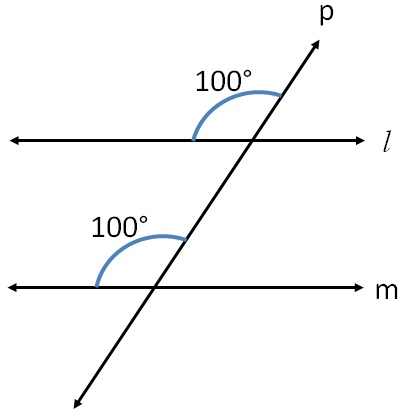-a-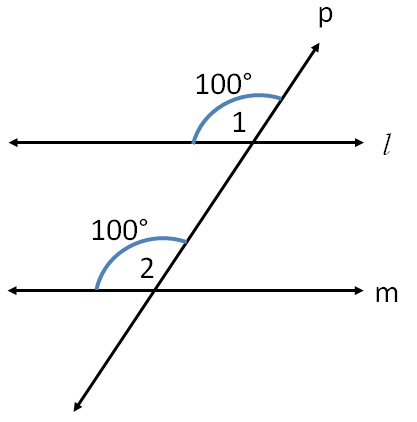Here,

∠1 = ∠2 = 100°

For lines l & m,

With transversal p

∠1 & ∠2 are corresponding angles.

And they are equal.

So, lines l & m are parallel

-ea-

Is l m ?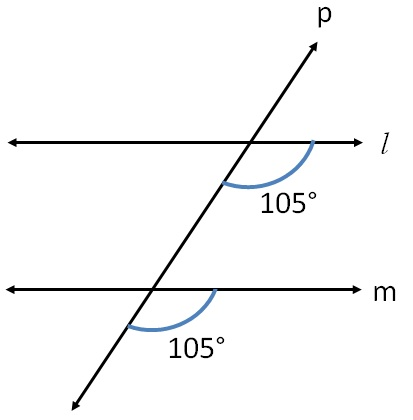-a-

Here,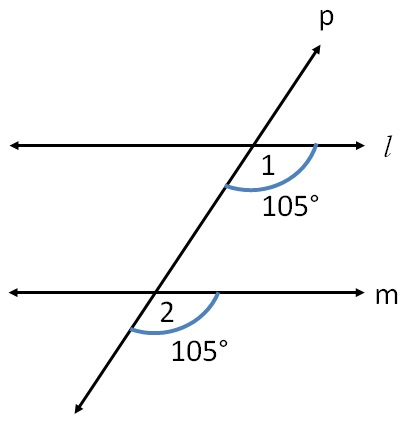Here,

∠1 = ∠2 = 105°

For lines l & m,

With transversal p

∠1 & ∠2 are corresponding angles.

And they are equal.

So, lines l & m are parallel

-ea-

Is l m ?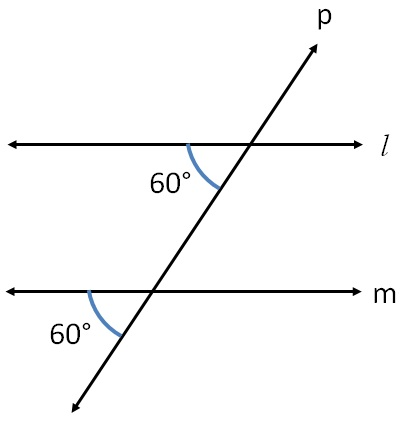-a-

Here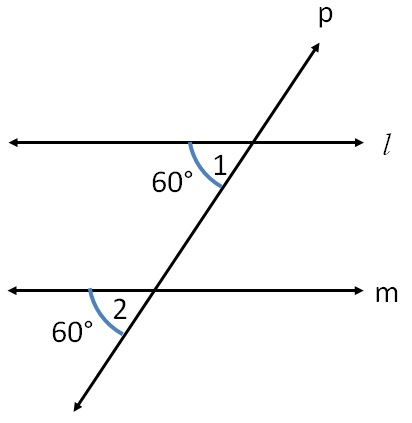Here,

∠1 = ∠2 = 60°

For lines l & m,

With transversal p

∠1 & ∠2 are corresponding angles.

And they are equal.

So, lines l & m are parallel

-ea-

Is l m ?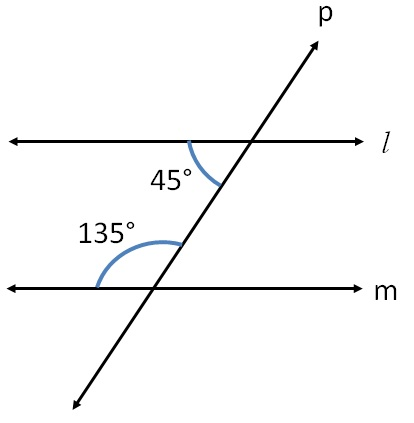-a-

Here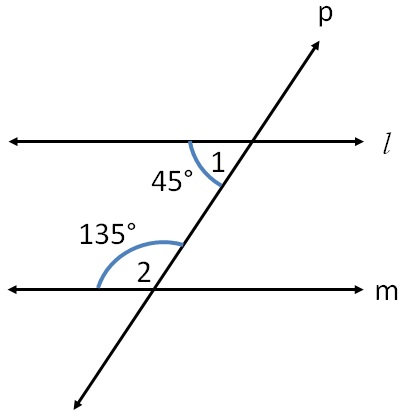Here,

∠1 + ∠2 = 45° + 135°

= 180°

For lines l & m,

With transversal p

∠1 & ∠2 are interior angles on the same side of transversal

And they are supplementary

So, lines l & m are parallel

-ea-

Is l ∥ m ?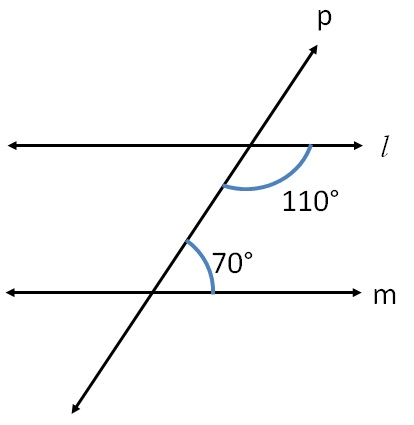-a-

Here,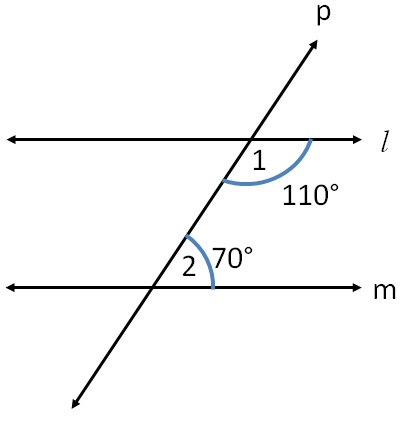Here,

∠1 + ∠2 = 110° + 70°

= 180°

For lines l & m,

With transversal p

∠1 & ∠2 are interior angles on the same side of transversal

And their sum is 180°.

So, they are supplementary

So, lines l & m are parallel

-ea-

Is l m ?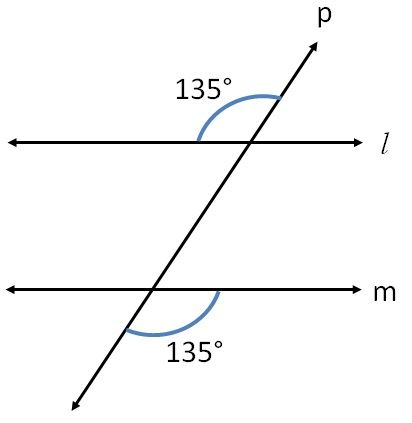-a-

Here,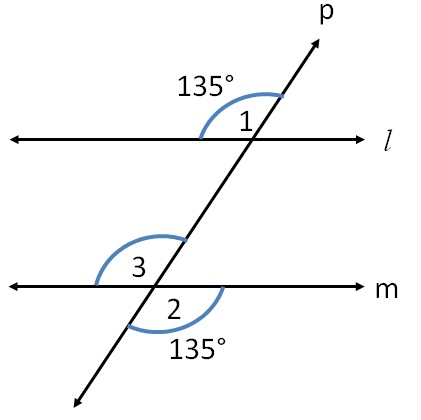Here,

∠3 = ∠2                    (Vertically opposite angles)

∠3 = 135°

Now, ∠1 = ∠3 = 135°

For lines l & m,

With transversal p

∠1 & ∠3 are corresponding angles.

And they are equal.

So, lines l & m are parallel

-ea-

Is l m ?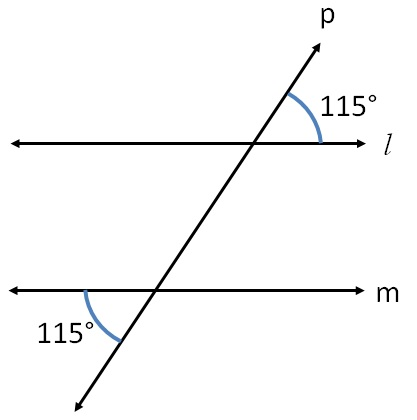-a-

Here,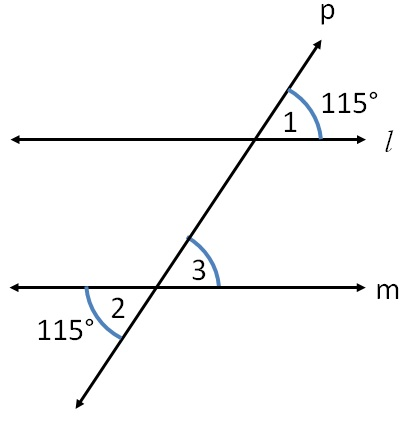Here,

∠3 = ∠2                     (Vertically opposite angles)

∠3 = 115°

Now, ∠1 = ∠3 = 115°

For lines l & m,

With transversal p

∠1 & ∠3 are corresponding angles.

And they are equal.

So, lines l & m are parallel

-ea-

Is l m ?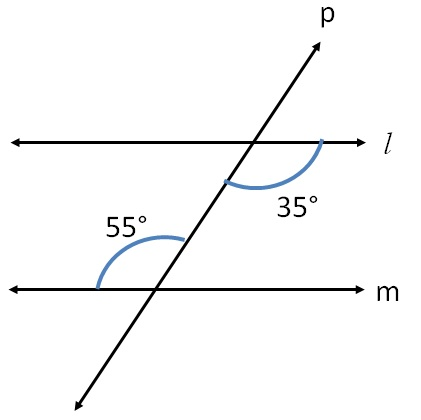-a-

Here,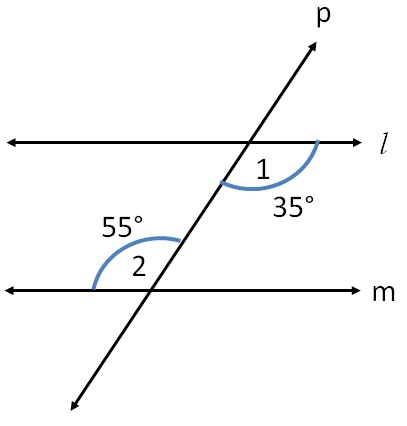Here,

∠1 ≠ ∠2

For lines l & m,

With transversal p

∠1 & ∠2 are alternate interior angles.

And they are not equal.

So, lines l & m are not parallel

-ea-

Is l m ?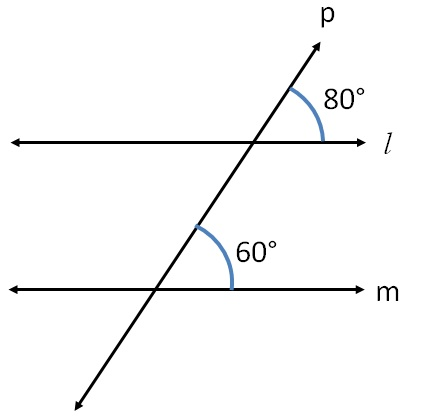-a-

Here,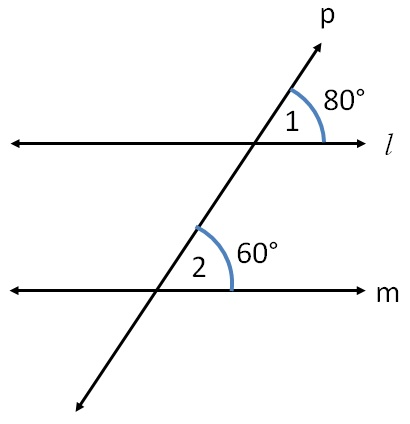Here,

∠1 ≠ ∠2

For lines l & m,

With transversal p

∠1 & ∠2 are corresponding angles.

But they are not equal.

So, lines l & m are not parallel

-ea-

Is l m ?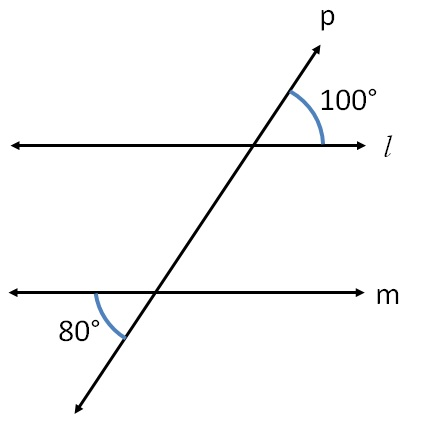-a-

Here,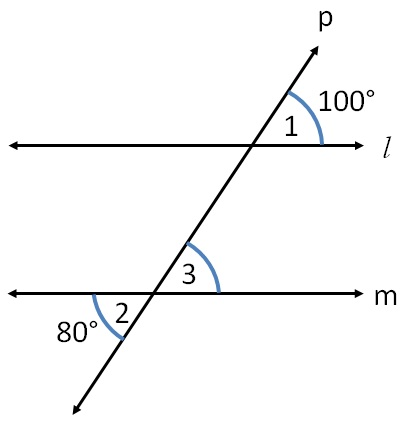Here,

∠3 = ∠2             (Vertically opposite angles)

∠3 = 80°

≠ 100°

∴ ∠1 ≠ ∠3

For lines l & m,

With transversal p

∠1 & ∠3 are corresponding angles.

But they are not equal.

So, lines l & m are not parallel

-ea-

Learn in your speed, with individual attention - Teachoo Maths 1-on-1 Class